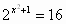# Translations of Encyclopedia about Mathematics

## Exponential Equations

Exponential equations and inequalities are equations or inequalities in which a variable appears at least once as an exponent.

Examples:

a)b)We can quickly substitute a test for (a), based on the solution x = 4, but, for (b), substituting a test does not help. In this case, it is necessary to use a logarithm and to solve a logarithmisation of both sides by "fishing out" the equation x2 + 1 in order to get a normal equation.

Growth and Decay, Examples of Using Exponential Functions

Growth or decay are changes in one quantity, growth quantities, over time. We can observe such growths in many areas, such as biological growth over some area, the growth of populations, the growth of a colony of yeast, the mortification of forests, radioactive decay in physics, or the growth of the consumer price index during inflation.

It is not possible to make exact predictions over longer periods of times because we derive our predictions based on what we know and, over time, other factors may arise which might influence the outcome. To simulate growth, it is necessary to formulate a function which depicts growth best describing reality.

Exponential functions are suitable for describing such growth processes and for modeling them.

Translating Dutch Hungarian Translations Hungarian Dutch Translating Swedish Czech Translations Czech Swedish Translating RussianEnter your search terms Submit search form